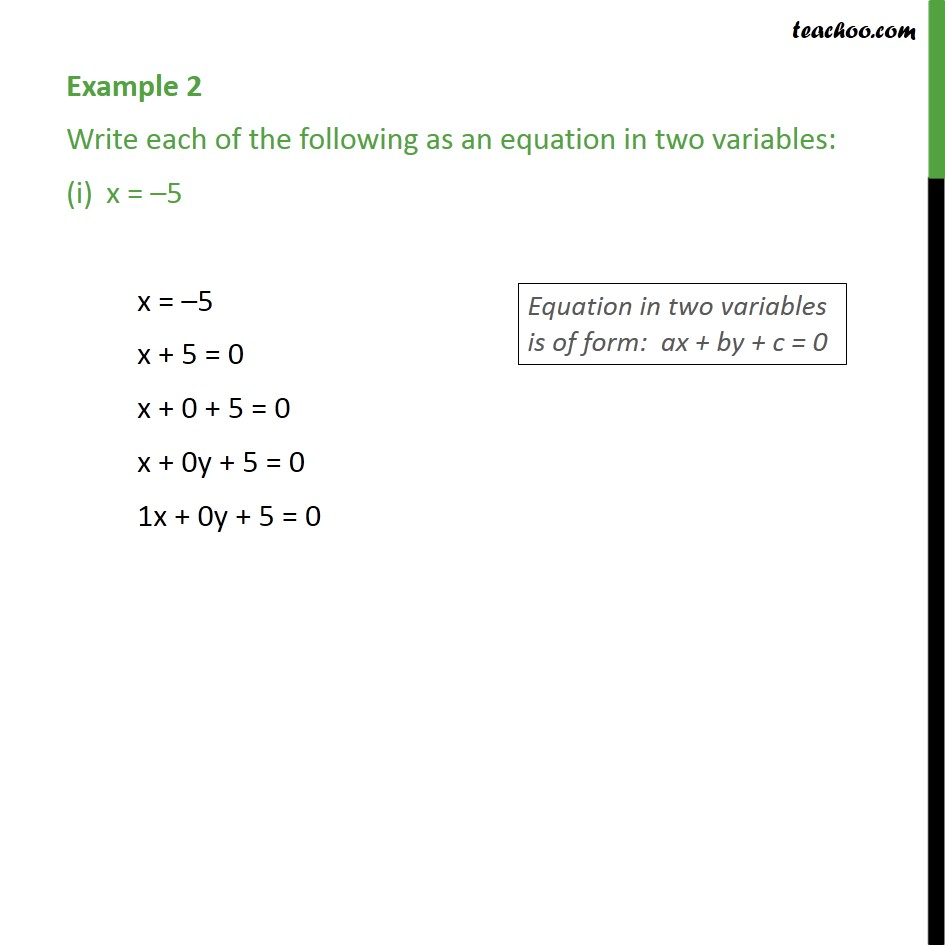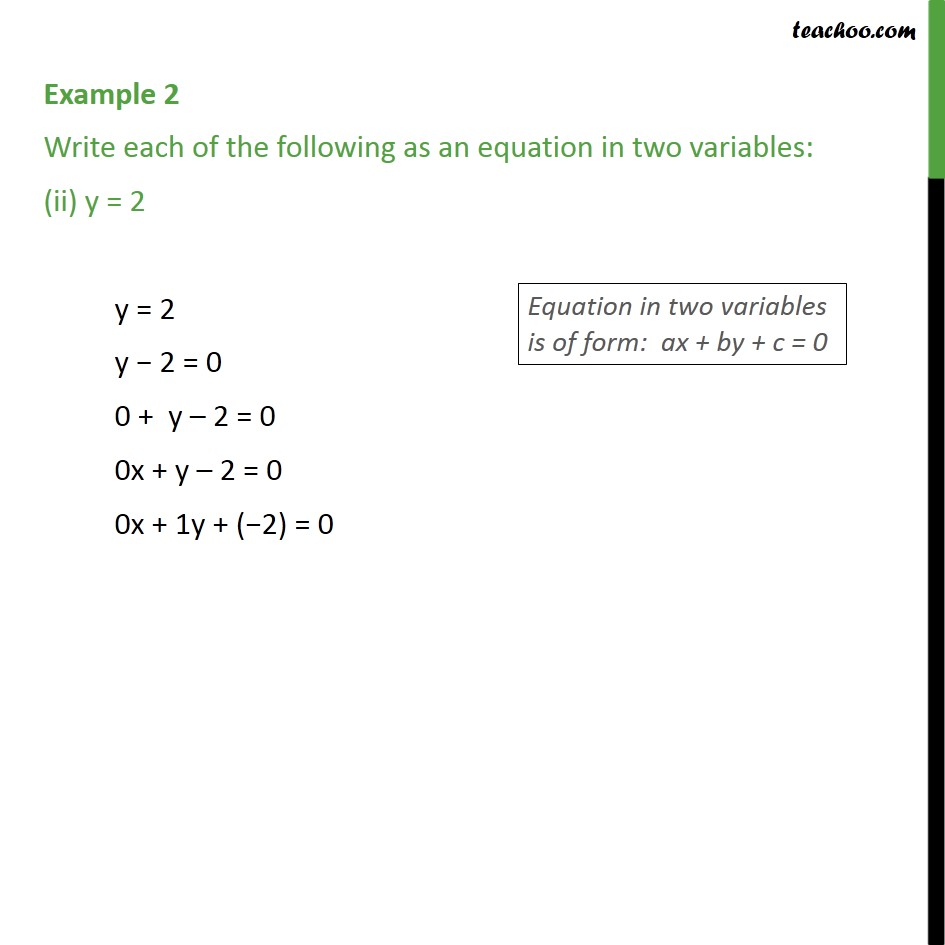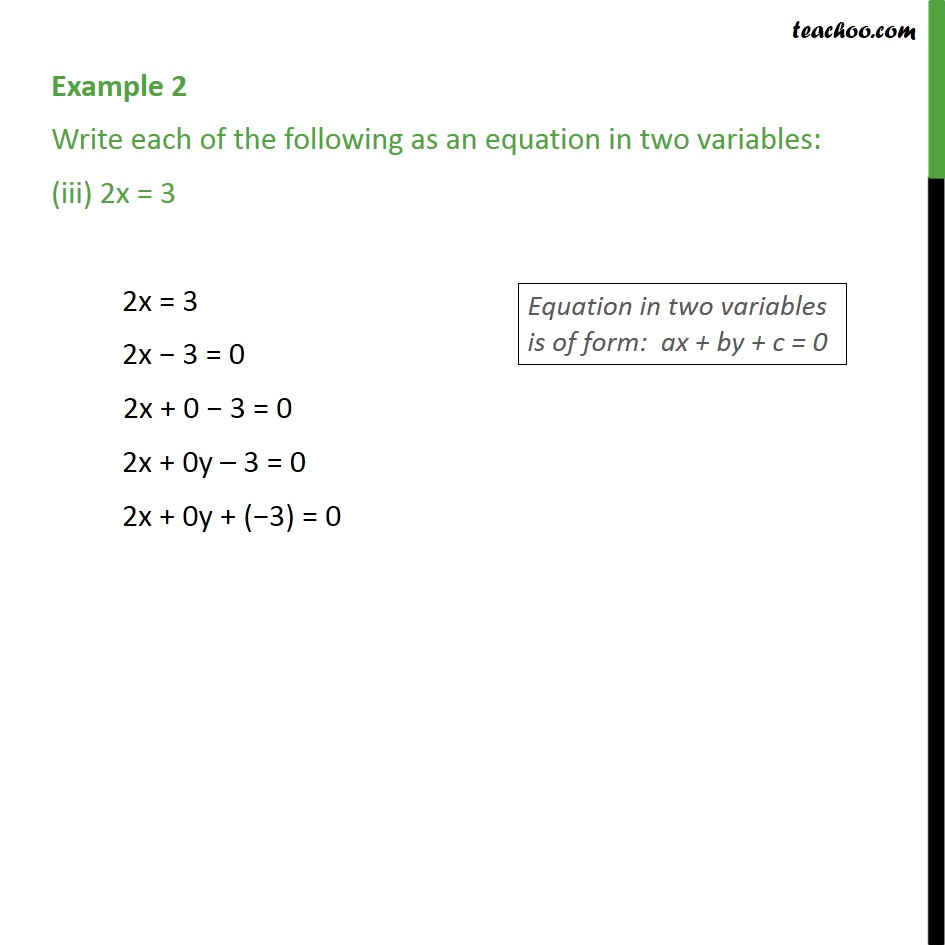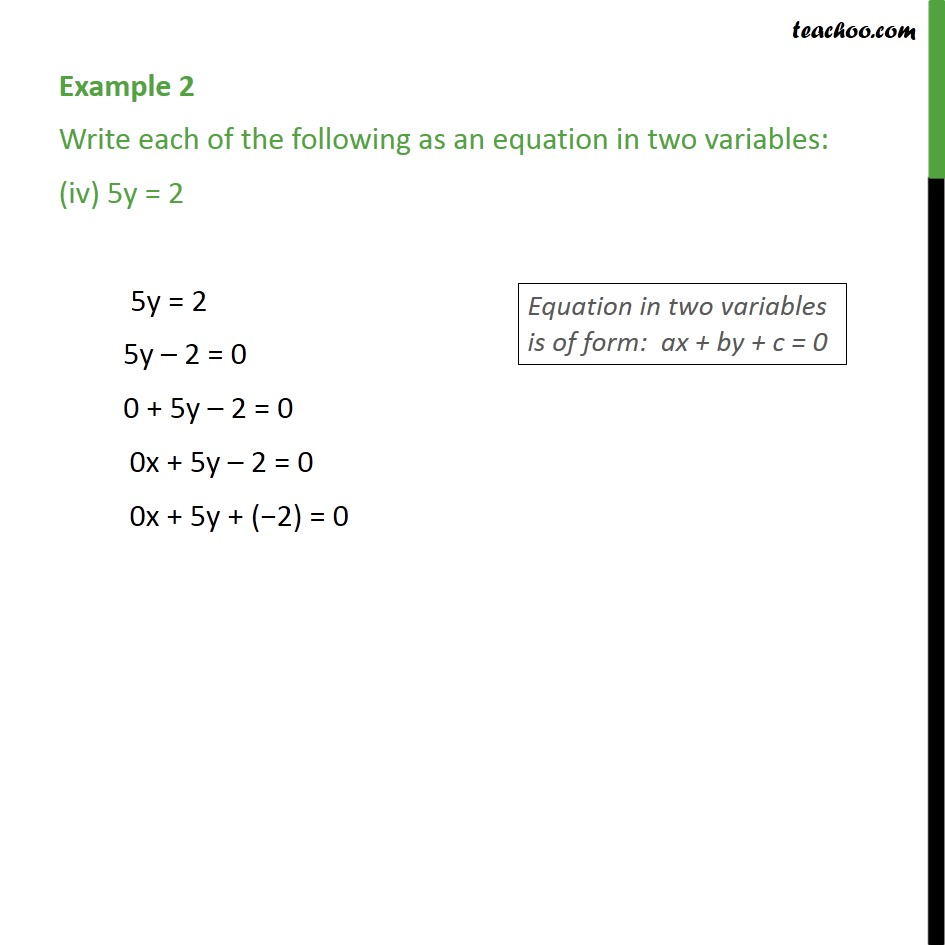Subscribe to our Youtube Channel - https://you.tube/teachoo

1. Class 9
2. Important Questions for Exam - Class 9
3. Chapter 4 Class 9 Linear Equations in Two Variables

Transcript

Example 2 Write each of the following as an equation in two variables: x = 5 x = 5 x + 5 = 0 x + 0 + 5 = 0 x + 0y + 5 = 0 1x + 0y + 5 = 0 Example 2 Write each of the following as an equation in two variables: (ii) y = 2 y = 2 y 2 = 0 0 + y 2 = 0 0x + y 2 = 0 0x + 1y + ( 2) = 0 Example 2 Write each of the following as an equation in two variables: (iii) 2x = 3 2x = 3 2x 3 = 0 2x + 0 3 = 0 2x + 0y 3 = 0 2x + 0y + ( 3) = 0 Example 2 Write each of the following as an equation in two variables: (iv) 5y = 2 5y = 2 5y 2 = 0 0 + 5y 2 = 0 0x + 5y 2 = 0 0x + 5y + ( 2) = 0

Chapter 4 Class 9 Linear Equations in Two Variables

Class 9
Important Questions for Exam - Class 9